# Difference between revisions of "Compact times paracompact implies paracompact"

This article states and proves a result of the following form: the product of two topological spaces, the first satisfying the property Compact space (?) and the second satisfying the property Paracompact space (?), is a topological space satisfying the property Paracompact space (?).
View other such computations

## Statement

### Verbal statement

The product of a compact space with a paracompact space (given the product topology), is paracompact

### Statement with symbols

Let$X$ be a compact space and$Y$ a paracompact space. Then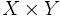$X \times Y$ is paracompact.

## Related facts

Other results using the same proof technique:

## Facts used

1. Tube lemma: If$X$ is a compact space and$Y$ is a topological space. Then, given any open subset$U$ of$X \times Y$ containing$X \times \{ y \}$ for some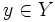$y \in Y$, there exists an open subset$V$ of$Y$ such that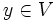$y \in V$ and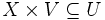$X \times V \subseteq U$.

## Proof

Given: A compact space$X$, a paracompact space$Y$.

To prove If$U_i$ form an open cover of$X \times Y$, there exists a locally finite open refinement of the$U_i$.

Proof:

1. For any point$y \in Y$, there is a finite collection of$U_i$ that cover$X \times \{ y \}$: Since$X$ is compact, the subspace$X \times \{ y \}$ of$X \times Y$ is also compact, so the cover by the open subsets$U_i$ has a finite subcover.
2. Let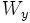$W_y$ be the union of this finite collection of open subsets$U_i$. By fact (1), there exists an open subset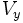$V_y$ of$Y$ such that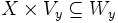$X \times V_y \subseteq W_y$.
3. The$V_y$ form an open cover of$Y$.
4. There exists a locally finite open refinement, say$\mathcal{P}$ of the$V_y$ in$Y$: This follows from the fact that$Y$ is paracompact.
5. We can construct a locally finite open refinement of$U_i$ from these:
1. For each member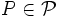$P \in \mathcal{P}$, there exists$V_y$ such that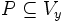$P \subseteq V_y$. Thus,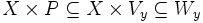$X \times P \subseteq X \times V_y \subseteq W_y$.$W_y$, in turn, is a union of a finite collection of$U_i$s. Thus,$X \times P$ is the union of the intersections$(X \times P) \cap U_i$.
2. Since the$X \times P$ together cover$X \times Y$, the$(X \times P) \cap U_i$ are an open cover of$X \times Y$ that refines the$U_i$s.
3. Finally, we argue that$(X \times P) \cap U_i$ is a locally finite open cover: Suppose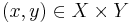$(x,y) \in X \times Y$. Since$\mathcal{P}$ is a locally finite open cover of$Y$, there exists an open subset$Q$ of$Y$ containing$y$ such that$Q$ intersects only finitely many members of$\mathcal{P}$. Thus, the neighborhood$X \times Q$ intersects only finitely many$X \times P$s, which in turn give rise to finitely many$(X \times P) \cap U_i$s each. Thus,$X \times Q$ intersects only finitely many members of the open cover.